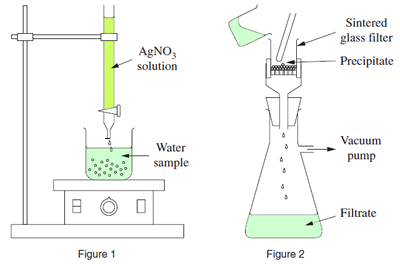Gravimetric exercises (NSW 2007) The diagrams, on the right, represent equipment used in an investigation to determine the chloride ion concentration in a water sample. Atomic mass Ag=107.9, Na=23.0, Cl=35.5, N=14.0, O=16.0a) Describe how you could, using the equipment in the diagram, determine the chloride ion concentration in a water sample. Include a balanced equation. . Click here for solutionb) If the volume of the water sample being tested is 50.0 mL and the mass of the dried precipitate obtained is 3.65 g, calculate the chloride ion concentration in the water sample in ppm. Click here for solutionc) Why is it important to determine the chloride ion concentration in water? Click here for solution